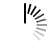# Travelling Salesman Problem

One sales-person is in a city, he has to visit all other cities those are listed, the cost of traveling from one city to another city is also provided. Find the route where the cost is minimum to visit all of the cities once and return back to his starting city.

The graph must be complete for this case, so the sales-person can go from any city to any city directly.

Here we have to find minimum weighted Hamiltonian Cycle.

#### Input and Output

```Input:
Cost matrix of the matrix.
0  20 42 25 30
20  0 30 34 15
42 30  0 10 10
25 34 10  0 25
30 15 10 25  0

Output:
Distance of Travelling Salesman: 80```

#### Algorithm

`travellingSalesman (mask, pos)`

There is a table dp, and VISIT_ALL value to mark all nodes are visited

Input: mask value for masking some cities, position.

Output: Find the shortest route to visit all the cities.

```Begin
if mask = VISIT_ALL, then //when all cities are visited
return cost[pos, 0]
if dp[mask, pos] ≠ -1, then
finalCost := ∞

for all cities i, do
tempMask := (shift 1 left side i times)
if mask AND tempMask = 0, then
tempCpst := cost[pos, i] +
finalCost := minimum of finalCost and tempCost
done

dp[mask, pos] = finalCost
return finalCost
End```

#### Source Code (C++)

```#include<iostream>
#define CITY 5
#define INF 9999
using namespace std;

int cost[CITY][CITY] = {
{0, 20, 42, 25, 30},
{20, 0, 30, 34, 15},
{42, 30, 0, 10, 10},
{25, 34, 10, 0, 25},
{30, 15, 10, 25, 0}
};

int VISIT_ALL = (1 << CITY) - 1;

int dp;    //make array of size (2^n, n)

int travellingSalesman(int mask, int pos) {
if(mask == VISIT_ALL)    //when all cities are marked as visited
return cost[pos];    //from current city to origin

if(dp[mask][pos] != -1)    //when it is considered

int finalCost = INF;

for(int i = 0; i<CITY; i++) {
if((mask & (1 << i)) == 0) {    //if the ith bit of the result is 0, then it is unvisited
int tempCost = cost[pos][i] + travellingSalesman(mask | (1 << i), i);    //as ith city is visited
finalCost = min(finalCost, tempCost);
}
}
return dp[mask][pos] = finalCost;
}

int main() {
int row = (1 << CITY), col = CITY;
for(int i = 0; i<row; i++)
for(int j = 0; j<col; j++)
dp[i][j] = -1;    //initialize dp array to -1
cout << "Distance of Travelling Salesman: ";
cout <<travellingSalesman(1, 0);    //initially mask is 0001, as 0th city already visited
}```

#### Output

`Distance of Travelling Salesman: 80`